What is Elevation in boiling point?

The boiling of a liquid may be defused by the temperature at which its vapour pressure which is equal to atmospheric pressure. The effect of addition in a non-volatile solute on the boiling point shown and its solution containing non-volatile solute with temperature are represented by the curves AB and CD respectively. It is evident by the curves temperature the vapour pressure of solutions is lower than that of the pure solvent and thus, the vapour pressure.

Curve for solution runs below that of the pure solvent. At temperature T0, the vapour pressure of the pure solvent becomes equal to the atmospheric pressure. Thus, the atmospheric pressure and therefore, it is necessary to heat the solution to a higher temperature sayT1 in the atmospheric pressure. Thus, it is clear that the solution in higher temperature than the pure solvent. Evidence T1 - T0 (or Δ Tb)is the elevation in boiling point vapour pressure (Δp), the elevation in the boiling point is also proportional to the solute concentration. Thus,

ΔTb ∝ Δp

According to Raoult's law, Δp ∝ xB

∴ ΔTb ∝ xB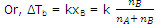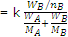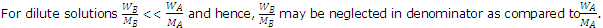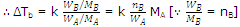If WA is the mass of solvent in kg, then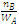is equal to molality (m) of the solution

ΔTb = kMA m

Here, k and MA are constants and hence their product, i.e. kMA is replaced by another constant K2.

ΔTb = Kb m, where Kb is called boiling point-elevation constant or molal elevation constant or molal ebullioscopic constant.

As elevation in boiling point depends upon the relative number of moles of solute and solvent but does not depend upon the nature of solute, so it is a colligative property.

#### Related Questions in Chemistry

Normal 0

• ##### Q :Quastion of finding vapour pressure

Vapour pressure of CCl425Degree C at is 143mm of Hg0.5gm of a non-volatile solute (mol. wt. = 65) is dissolved in 100ml CCl4 .Find the vapour pressure of the solution (Density of CCl4 = = 1.58g /cm2): (a)141.43mm (b)

• ##### Q :Mass percent Help me to go through this

Help me to go through this problem. 10 grams of a solute is dissolved in 90 grams of a solvent. Its mass percent in solution is : (a) 0.01 (b) 11.1 (c)10 (d) 9

• ##### Q :Describe characteristics of halides and

Halides characteristics

• ##### Q :Problem on distribution law The

The distribution law is exerted for the distribution of basic acid among: (i) Water and ethyl alcohol (ii) Water and amyl alcohol (iii) Water and sulphuric acid (iv) Water and liquor ammonia What is the right answer.

• ##### Q :What is synthetic rubber and how it

To meet human needs, scientists have started preparing synthetic rubbers. Besides having similar properties as natural rubbers they are tougher, more flexible and more durable than natural rubber. They are capable of getting stretched to twice its length. Though, it reverts to its original shape

• ##### Q :Number of moles of potassium chloride

Choose the right answer from following. The number of moles of KCL in 1000ml of 3 molar solution is: (a)1 (b)2 (c)3 (d)1.5

• ##### Q :Means of molal solution Choose the

Choose the right answer from following. A molal solution is one that contains one mole of a solute in: (a) 1000 gm of the solvent (b) One litre of the solvent (c) One litre of the solution (d) 22.4 litres of the solution